“Relationships suck” — Everyone at some point in their life

### What is a relation?#

There is a relation between two things if there is some connection between them. Relations exist on Facebook, for example. In this blog post we’ll be studying relations between sets.

Believe it or not, but relations between sets occur naturally in every day life such as the relation between a company and its telephone numbers

### The Cartesian Product#

An ordered pair contains 2 items such as (1, 2) and the order matters. (1, 2) is not equal to (2, 1) unlike in set theory. Sets of ordered pairs are called binary relations.

Let A and B be sets then the binary relation from A to B is a subset of A x B. In other words, a binary relation from A to B is a set of ordered pairs where the first element of each ordered pair comes from A and the second from B.

So a binary relation such as A = {a, b} and B = {1, 2} would be A x B = { (a, 1), (b, 1), (a, 2), (b, 2) }

Note: (a, b) = (c, d) if and only if a = c and b = d.

A relation between A and B is always a subset of the cartesian product.

Examples of the cartesian product are cartesian coordinates, created by Decartes or friendships on Facebook or Twitter.

The Cartesian Product can be used to create sets too such as the below mathematical formula:

A =  {1, 3, 5, 7} B = {2, 4, 6}{(X, Y) ∈A x B | X + Y = 9}{(3, 6), (2, 7), (5, 4)}

An interesting fact about the cartesian product is that the cardinality of A + the cardinality of B is how many items will be in the cartesian product. |A| + |B| = |Cartesian Product| unless the cartesian product has a filter applied to it like the above example.

#### Directed Graphs#

A directed graph is a graph with nodes connected by lines that have a direction attached to them, often called Diagraphs.

Let A and B be two finite sets and R a binary relation between them.

We represent the two sets as vertices (or nodes) on the graph.

For each binary relation (a, b) we draw an arrow linking the related elements.

Let’s create an example.

Set A = {1, 2} Set B = {3, 4} Let’s create a binary relation. A x B = {(1, 3), (2, 3), (2, 3), (2, 4)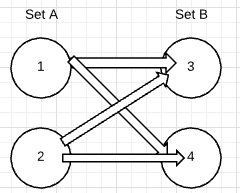A binary relation between set A and itself (A x A) is every possible combination of ordered pair, unless specified otherwise or a predicate is applied.

For example, the relation A x A where A is {1, 2} is

{(1, 2), (2, 1), (1, 1), (2, 2)}

If a set exists such as {1, 2, 3, 4} and there is a relation, R, on the set such that {y, x| y > x} then the set would be {(2, 1), (3, 2), (4, 3), (3, 1), (4, 1), (4, 2), (3, 1)}.

The ordered pair is always in the order specified, above it is specified as (y, x) and the relation only contains ordered pairs where the first element is larger than the second element in the ordered pair.

### Functions as Relations#

If 2 sets hold a relation such that for every element in set A there is a relation with at least one element in set B then that relation is said to be functional.

Example A = {1, 2} B = {3, 4, 5} Relation between A and B results in at least the ordered pairs of: {(1, 3), (2, 4)} Then the relation A x B is functional.

#### Inverse Relation#

Because a relation is said to be functional, we can steal a property from functions in mathematics, specifically calculating the inverse of a function.

In English, the inverse of a relation is the exact inverse of the set of ordered pairs of the original input.

Let’s look at the set {(0, 2), (3, 4), (-3, -2), (2, 4)}

To find the inverse of this relation, all we need to do is flip over the ordered pairs. So the inverse is

Inverse of original relation = { (2, 0), (4, 3), (-2, -3), (4, 2)}

### Composition of relations#

We can also create composition of relations. For example:

If R is the relation “is a sister of” and S is the relation “is a parent of” thenS◦R is the relation that “is an aunt of” and S◦S is the relation that “is a grandparent of”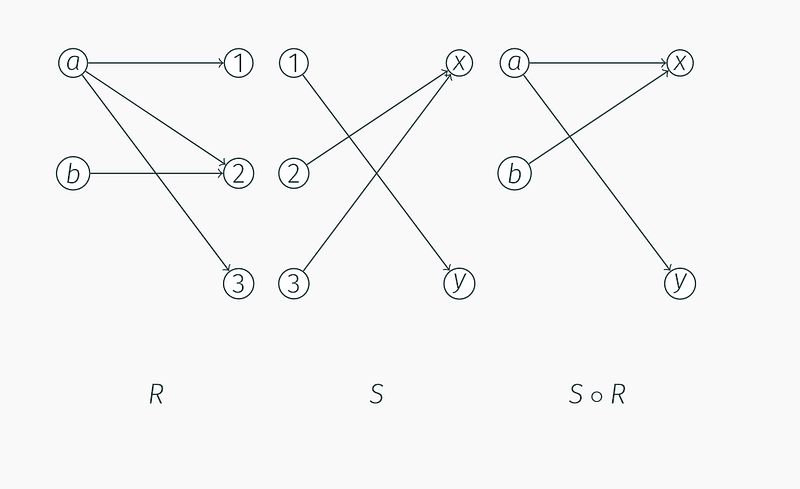DiaGraph representation, taken from Boris Konev’s Slides In the above picture, we can see that you can get to Y from A: a -> 1 -> y.

The diagraph on the right of the image is the composition of S and R.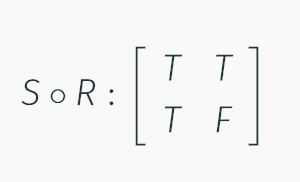Truth table of composition Knowing the diagraph, you can represent the composition in a truth table like above.

### Matrices#

“Unforunately, no one can be told what the Matrix is. You have to see it for yourself.” — Morpheus

Watch this video for an introduction to matrices and their origin from amazing Youtuber 3blue1brown

A matrix is an array used to represent data in Mathematics, Computer Science and Physics. Another way of representing a binary relation between two finite sets is to use a matrix. It is best to show this using an example:

A = {1, 3, 5, 7} B = {2, 4, 6} And given the relation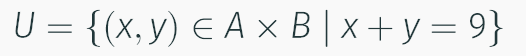Relation Then the matrix can be made like so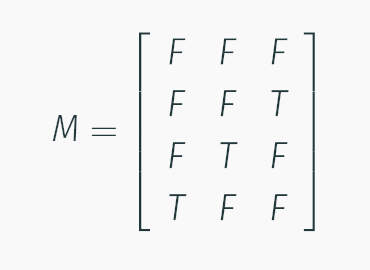Matrix The columns are labeled from set B and the rows are labeled from set A, as seen in the below picture: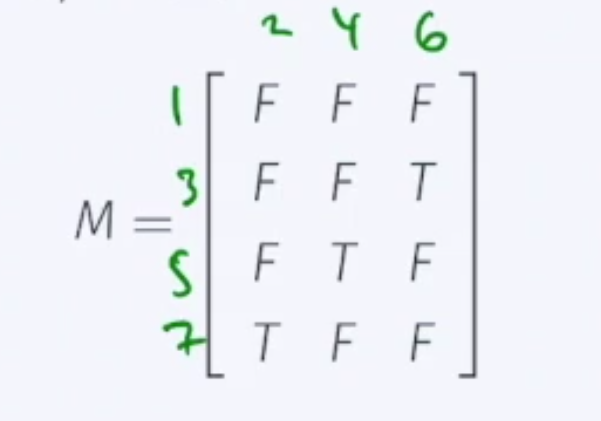Bad quality photo of a binary relation on a matrix If there exists a relation, we write True. Otherwise we write False. According to the above relation, 6 + 3 makes 9 which is in the relation, therefore it is True in the matrix.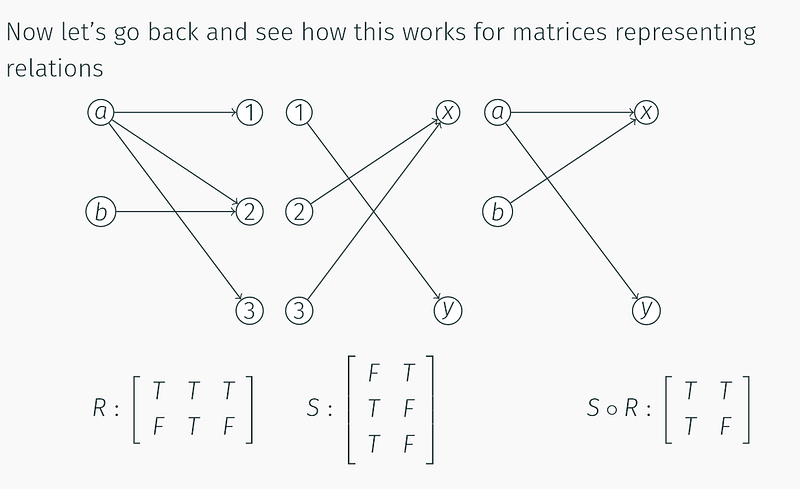This is literally me copying Boris’s slides and explaining them The relation R shows a matrix where the side on the left is the set {a, b} and the part on top is {1, 2, 3}. This shows that there is a relationship between a and 1 but no relationship between b and 1.

The second relation, S, is self-explanatory if you have read the previous paragraph.

The next relation is a composition of relations. There are 4 total elements over 2 sets in the diagraph, therefore the matrix contains 4 elements.

#### Logical Boolean Matrices#

A logical boolean matrix is a matrix which only has entries from the domain of Boolean Algebra, {0, 1} or {True, False}, the matrices seen above are logical boolean matrices.

### Properties of Binary Relations#

Binary relations can hold certain properties, in this we will explore them.

#### Infix Relation Symbols#

We can show a relationship using infix notation. Infix notation is notation that is placed in the middle of an equation, as compared to a relation outside of the notation.

An example of an infix notation is X < Y or 6 + 3 or X == Y. Infix notation doesn’t seem too bad, right?

#### Reflexive#

A relationship is called reflexive if xRx. In other words, if x is equal to x, or x == x then the relationship is reflexive.

Given the example

A relation R on A is reflexive if (x, x) ∈ *R for every x *∈ AA relation called R on set A is reflexive if for every ordered pair, (x, x) is an element of the relation and for every x is an element of the set.This may sound confusing so let’s continue with out example. So if A = {1, 2, 3, 4} Then the following are all **reflexive **

1. R = {(1, 1), (2,2), (3,3), (4,4)}
2. R = {(1, 1), (1, 2), (2,2), (3,3), (4,4)}
3. R = {(1, 1), (1, 3), (2,2), (2,3), (3,3), (4, 1), (4,4)}

The first relation, number 1, has a special name. It is the identity relation. Every set contains at least 1 ordered pair where every element, x, in the set is an ordered pair in the form (x, x).

Note the following:

1) R={(1,1),(2,2),(3,1),(4,4)} 2) R={(1,1)} 3) R={(1,1),(1,3),(1,4),(2,1),(2,2),(3,1),(3,3),(4,3)}

Are they reflexive?

Nope. 1 does not contain the ordered pair (3,3). 2 is missing almost all of the ordered pairs and 3 is missing the ordered pair (4, 4).

How would you show a relationship is reflexive using infix notation?

xRx is the answer.Symetrical relations can also be represented as diagraphs.

#### Symmetry#

Denoted as “xRy implies yRx” symmetry is where the cartesian product of 2 elements appears in the relation.

An example is best to show this:

Let S = {1,2,3}Let R be a relationship on S that produces pp = { (2, 1), (3,3), (3,1), (2,2),(1,1), (1,2), (1, 3)}Is P symmetric?

Yes, P is symmetric. This is because the cartesian product of the elements of set S {1, 3} appear in the list. (1, 3) appears in the list and so does (3, 1).

(3, 3) is also symmetric, as xRy and yRx where x = 3 and y = 3. This may sound confusing, but once you understand that (x, y) is equal to (3, 3) and that repition does matter in ordered pairs, that the first 3 may be equivalent to the second 3, they are still both seperate elements in the ordered pair. If this was a set, they would not be symmetric.

#### Anti-Symmetric#

Anti-symmetric is the opposite of symmetric. If (b, a) exists in the set of ordered tuples then (a, b) does not exist.

An example of this is {(1,2)}. This is not symmetric.

A relation cannot be symmetric and anti-symmetric at the same time and a relation can not be anti-symmetric and symmetric.

#### Transistive#

Tranisitive properties often appear in many disciplines of mathematics. The principle is that if A -> B and B -> C then A -> C.

In a set, given X, Y and Z as relations if X -> Y and Y -> Z then X -> Z.

### Equivalence Relations#

A binary relation is called an equivalence relation if it is reflexive, transitive and symmetric.

#### Closure of relations#

Given a relation, X, the relation X may or may not have properties that make it symmetric, transitive or reflexive. What are the minimum amount of elements needed to be added to to the relation to make the relation transistive, reflexive, or symmetric?

#### Question#

It is best to explore relations on your own then to read how relations work. Let’s try an example:

A = {1, 2, 3} R = {(1,1), (1,2), (1,3), (2,3), (3,1)} What is the transistive closure of the above relation?

### Partition of a set#

A partition of a set is a grouping of the set into smaller subsetsthat are non-empty in such a way that each element appears once and only once in the subset

### Totally Ordered Sets#

A totally ordered set is a relation on a set, X, such that it is antisymmetric and transistive. Numbers are considered totally ordered because two numbers are either equal to eachother or one is smaller.

### Partially Ordered Sets#

A partially ordered set is a set that indicates that for certain pairs of elements in the set, (x,y), x < y.

An example of this is something that you can’t exactly put a number on, like the size of a book. Some books can be ordered in size like so: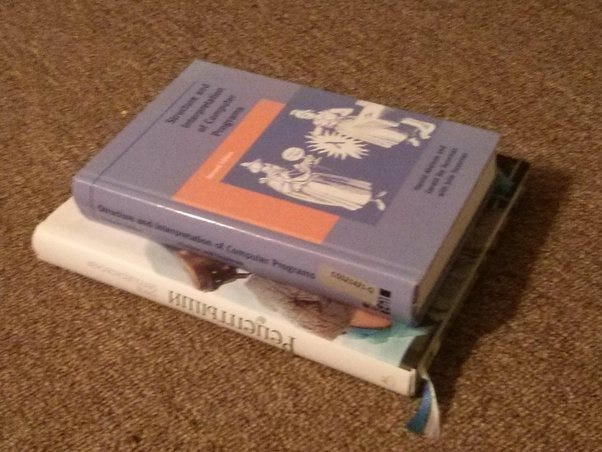But books have 2 dimensions, height and width. What if one book is taller but the other book has more width? What book is “larger” and what book is “smaller”?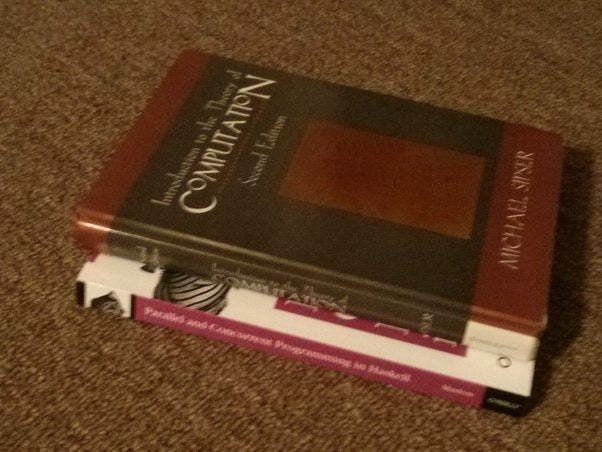This is where partial ordering comes in, we can order the pair in the first image but we cannot easily order the pair in the image directly above.